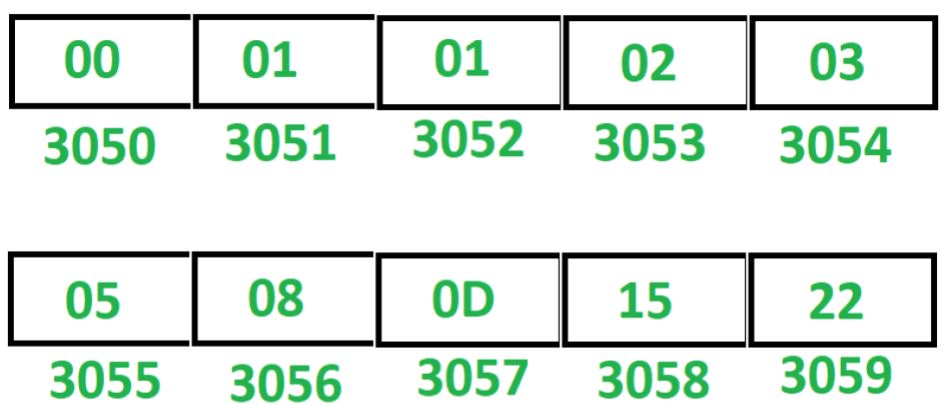Related Articles
8085 program to generate Fibonacci series
• Last Updated : 21 May, 2020

Problem – Write an assembly language program in 8085 microprocessor to generate Fibonacci series.

Example – Assume Fibonacci series is stored at starting memory location 3050.Note – This program generates Fibonacci series in hexadecimal numbers.

Algorithm –

1. Initialize register H with 30 and register L with 50, so that indirect memory M points to memory location 3050.
2. Initialize register B with 00, register C with 08 and register D with 01.
3. Move the content of B in M.
4. Increment M by 1 so that M points to next memory location.
5. Move the content of D in M.
6. Move the content of B in accumulator A.
7. Add the content of D in A.
8. Move the content of D in B.
9. Move the content of A in D.
10. Increment M by 1 so that M points to next memory location.
11. Move the content of A in M.
12. Decrements C by 1.
13. Jump to memory location 200C if ZF = 0 otherwise Halt the program.

Program –

MEMORY ADDRESSMNEMONICSCOMMENT
2000LXI H, 3050H <- 30, L <- 50
2003MVI C, 08C <- 08
2005MVI B, 00B <- 00
2007MVI D, 01D <- 01
2009MOV M, BM <- B
200AINX HM <- M + 01
200BMOV M, DM <- D
200CMOV A, BA <- B
200DADD DA <- A + D
200EMOV B, DB <- D
200FMOV D, AD <- A
2010INX HM <- M + 01
2011MOV M, AM <- A
2012DCR CC <- C – 01
2013JNZ 200CJump if ZF = 0
2016HLTEND

Explanation – Registers A, B, C, D, H, L are used for general purpose.

1. LXI H 3050: assigns 30 to H and 50 to L.
2. MVI B, 00: assigns 00 to B.
3. MVI C, 08: assigns 08 to C.
4. MVI D, 01: assigns 01 to D.
5. MOV M, B: moves the content of B in M.
6. INX H: increment M by 1.
7. MOV M, D: moves the content of D in M.
8. MOV A, B: moves the content of B in A.
9. ADD D: add the content of D and A. Store the result in A.
10. MOV B, D: moves the content of D in B.
11. MOV D, A: moves the content of A in D.
12. INX H: increment M by 1.
13. MOV M, A: moves the content of A in M.
14. DCR C: decrements C by 1.
15. JNZ 200C: jump to memory location 200C if ZF = 0.
16. HLT: stops executing the program and halts any further execution.

Attention reader! Don’t stop learning now. Get hold of all the important CS Theory concepts for SDE interviews with the CS Theory Course at a student-friendly price and become industry ready.

My Personal Notes arrow_drop_up